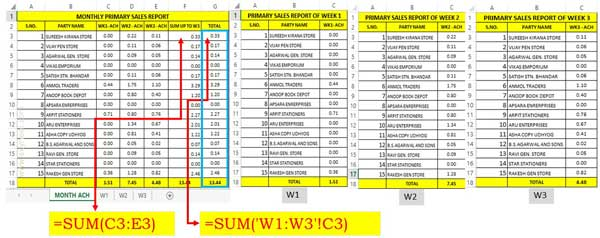# How to Apply formula on multiple worksheets with 3D reference in Excel

Excel allows you to apply formula on multiple worksheets with 3D reference. You can calculate the final result of different sheet values.

During working on an Excel you have to apply different functions on any worksheets which have the same body structure. But you have to face difficulty to calculate the accurate result. In this guide we have to discuss how to apply formula on multiple worksheets with 3D reference in Excel.

3D reference is an unique technique which is very useful for all users who are working on Excel to prepare different MIS reports. If you have more than one worksheet which have same the body structure and want to apply same function on these sheets then you have to face problem. But after reading this guide you can easily get an idea apply formula on multiple worksheets with 3D reference in Excel.

## How to Apply formula on multiple worksheets with 3D reference in Excel

Most of the Excel users does not aware about this trick. But it is an interesting and useful trick which helps you to solve your different problems. If you want to apply any function or formula on different sheets and want to view the result on any another sheet, then you have to face problem. But after reading this guide you can easily apply formula on multiple worksheets with 3D reference in Excel.

For eg. If you have three seprate worksheet’s in which you have weekly achievements which have same body structure & number of records. Now, you want to calculate the total of all weekly achievements of all different sheet’s values in another separate sheet. In that situation you have to take few given simple steps to apply formula on multiple worksheets with 3D reference in Excel.